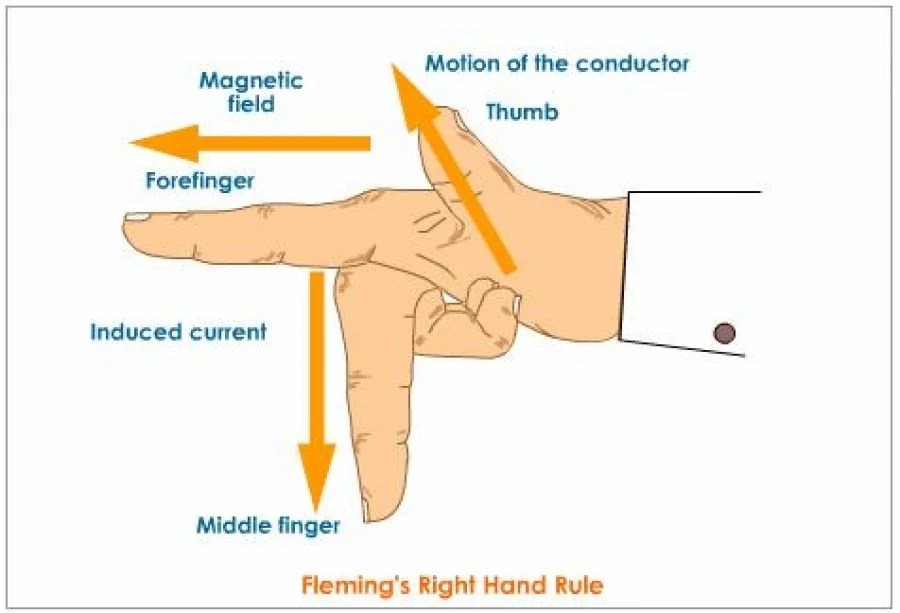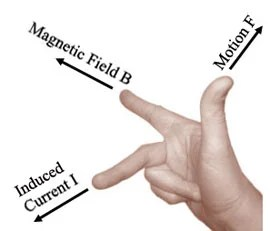# Fleming's Right Hand Rule

Rate this post

Introduction:

The fleming’s right hand rule is common rule and used in magnetic field in conductor. It is also used to convert the notations for vectors.The electromagnetism is most widely used technique and predict the current induction direction. Fleming’s right hand rule use the thumb, central and first finger for direction identification.

### Explanation for Fleming's Right Hand Rule

Right thumb rule in conductor:The thumb points along the direction of current, then the direction of the wrapped fingers will give the direction of magnetic field lines when we assume conductor carry the current in right hand.

The magnetic lines are anticlockwise when wire has current direction in upward.When the clockwise direction is performed in magnetic field the current is go in downward that is vertical axis.

### The finger indication:

The conductor motion direction is indicated by thumb and field direction is indicated by first finger and induced direction is indicated by second finger.

### Direction Association

Direction associated with ordered pair:

The ordered operation is use the fleming’s right hand rule. The two vectors a and b is horizontal to the vector c that is c is perpendicular to the vectors a and b.For example take the cross product, it defined by fleming’s right hand rule as `veca` x `vecb` = `vecc` .Direction associated with rotation:

This rotation operation use the different form of fleming’s right hand rule. The fingers are assign the rotation and magnetic field direction.The direction of rotation is indicated by thumb finger.

Applications for fleming’s right hand rule:

The cross product direction is evaluated by this rule.The following quantities are use the right hand rule:

• The rotating object’s angular velocity and rotational velocity.
• The force application at the point that is torque.
• Determine the direction of electric current and position in magnetic field.
• The causes of electric current in wire.
• The object velocity is determined by this fleming's right hand rule.

Fleming Right Hand Rule Video